Chapter 4 Part 1 - AD,AS and Related Concepts

Economics Class 12
Macroeconomics

## What is APC?

APC means Average Propensity to Consume

It is Ratio of aggregate consumption expenditure to aggregate income

Average Propensity to Consume = Consumption Expenditure/Income

APC = C/Y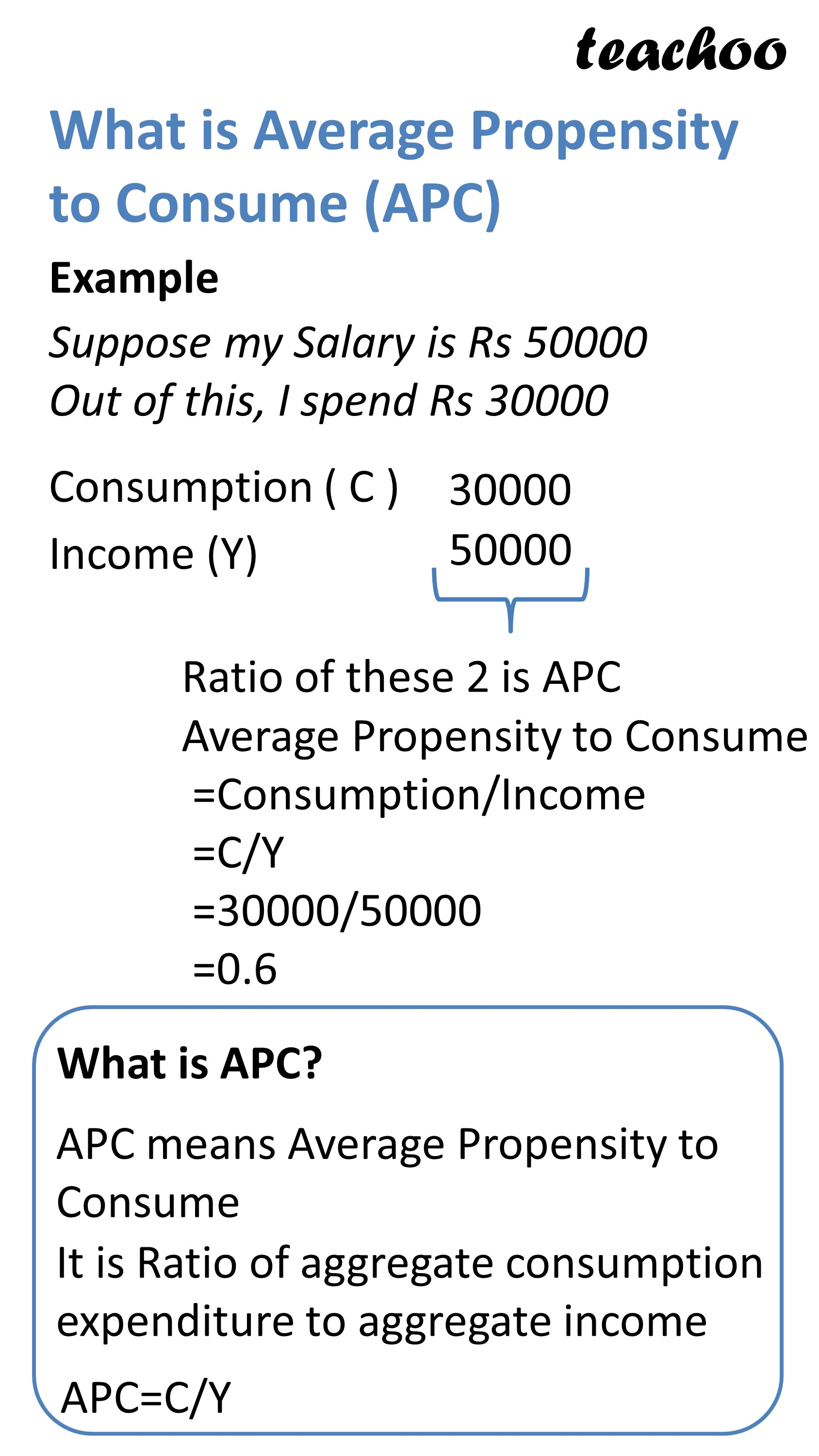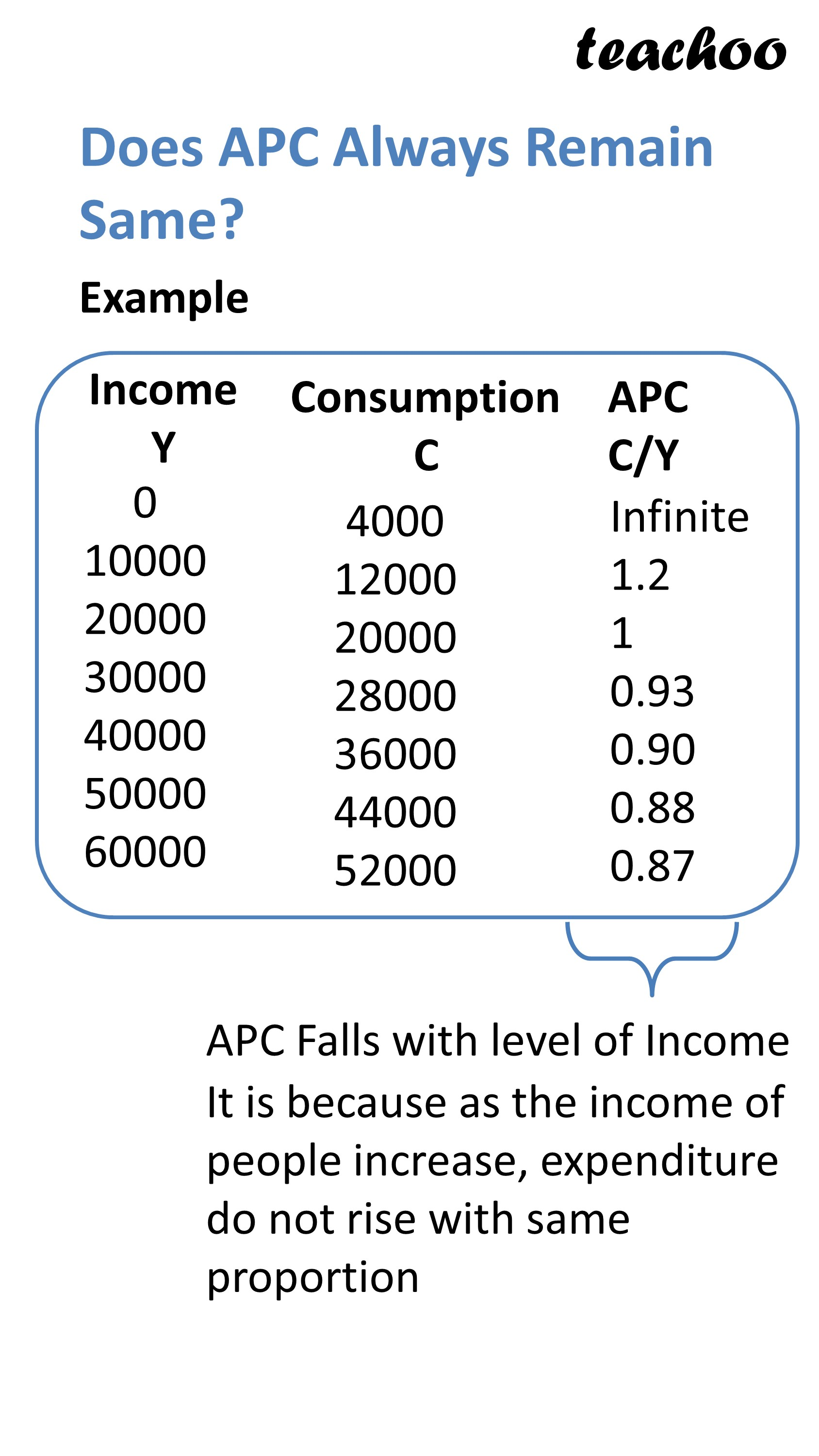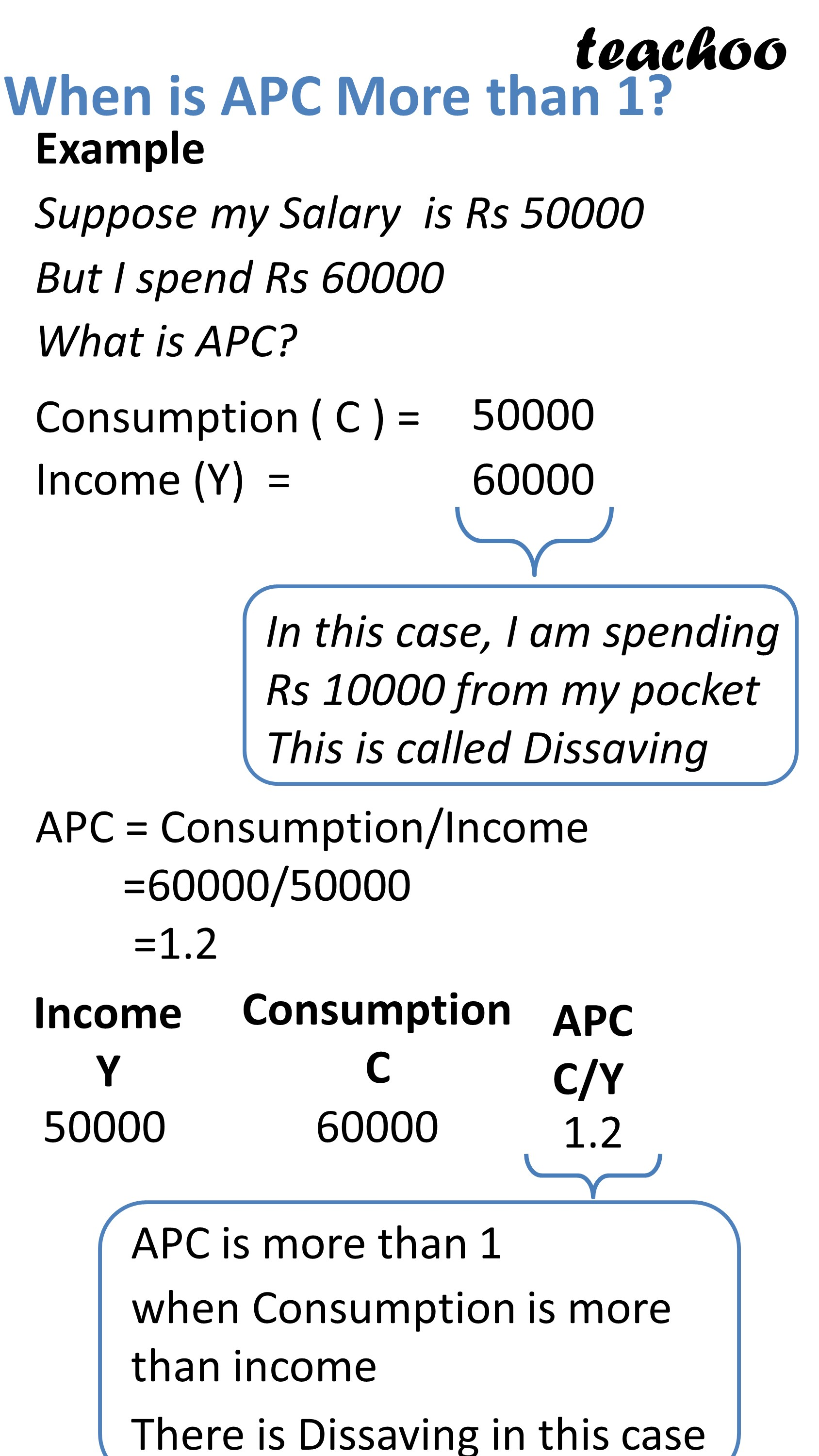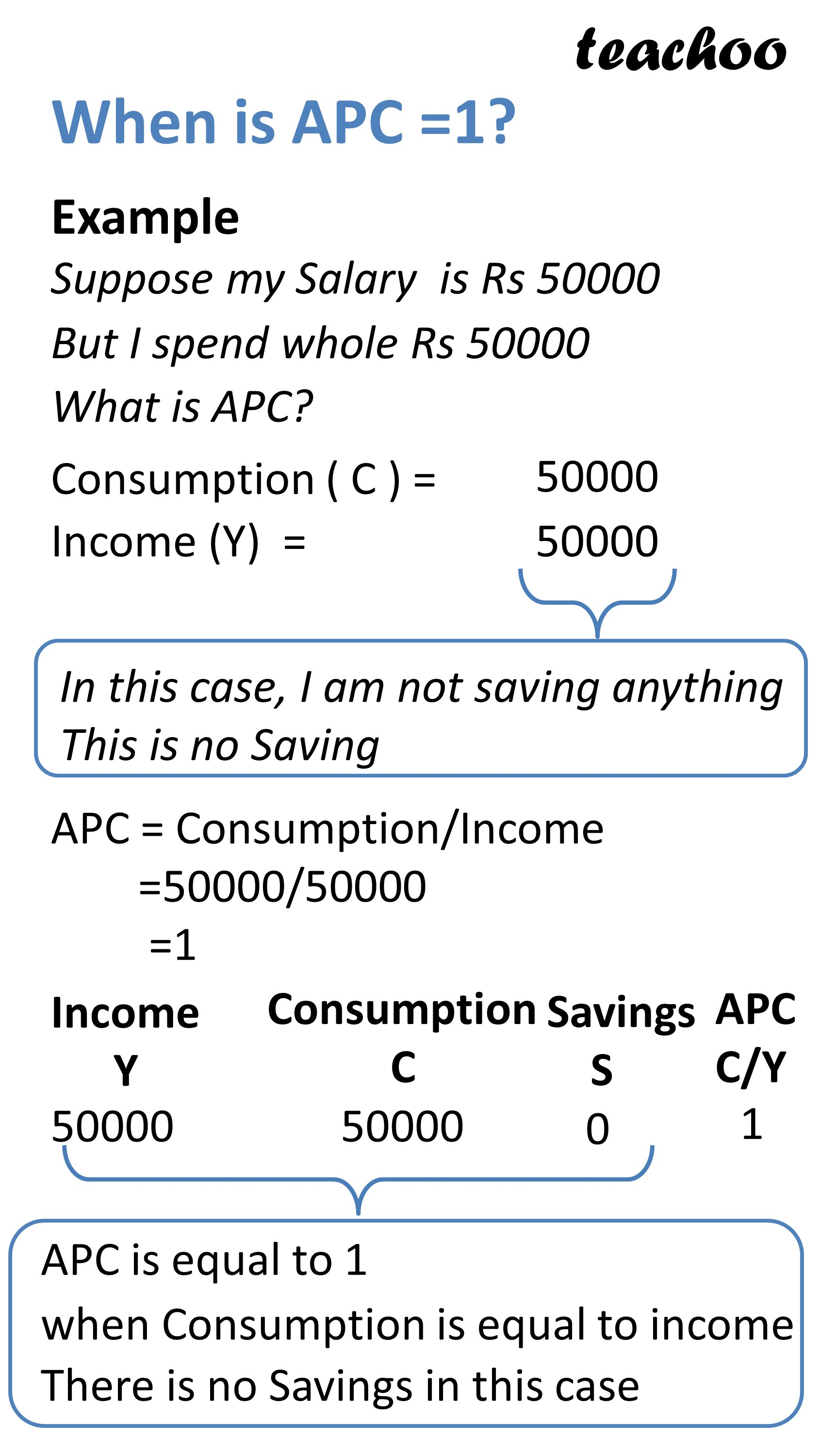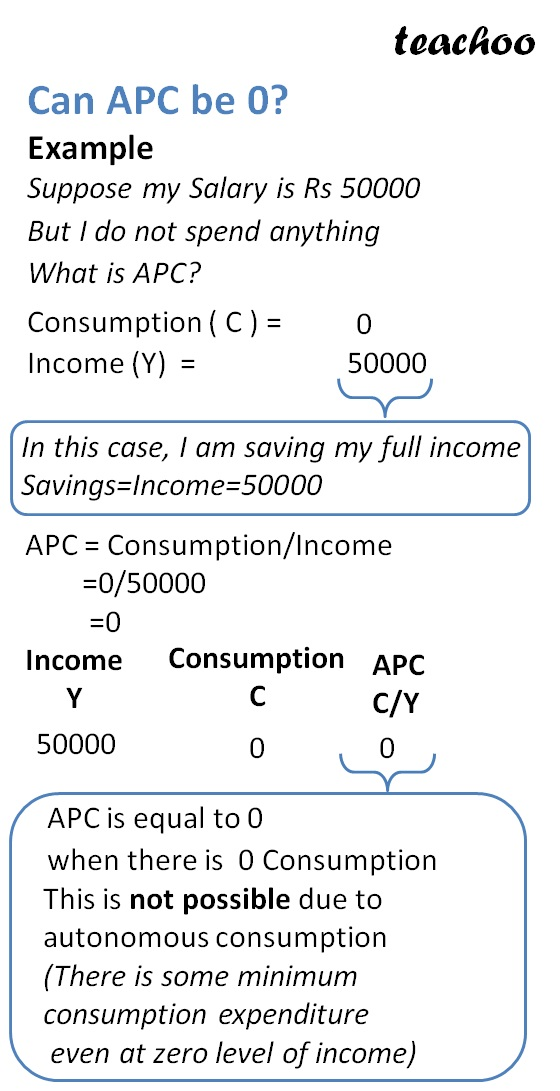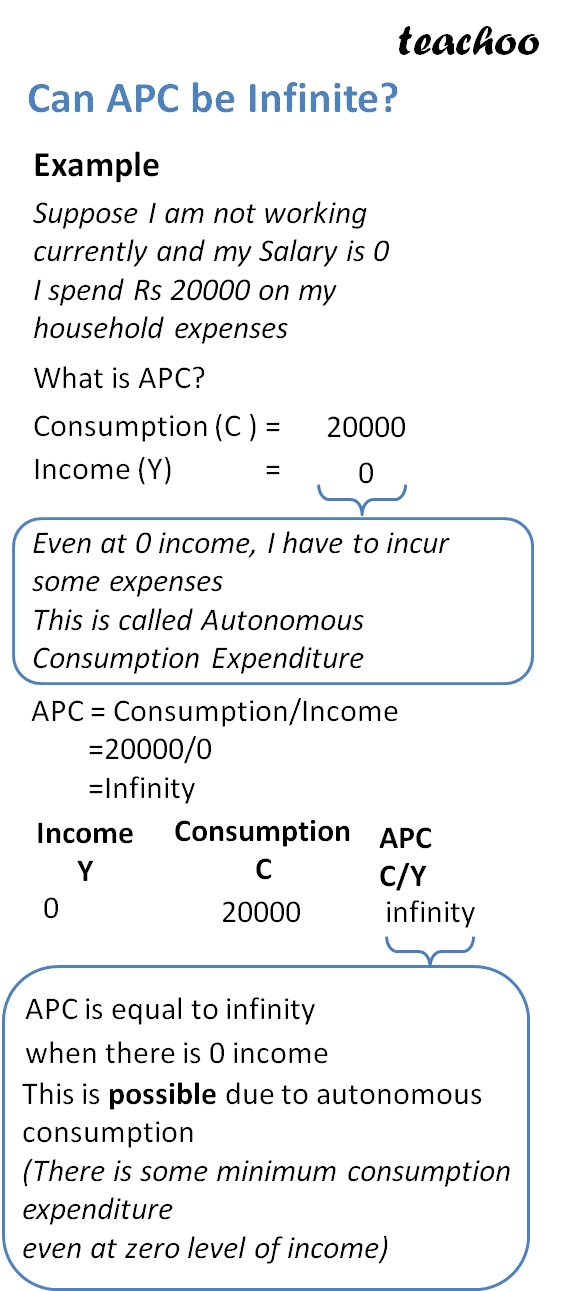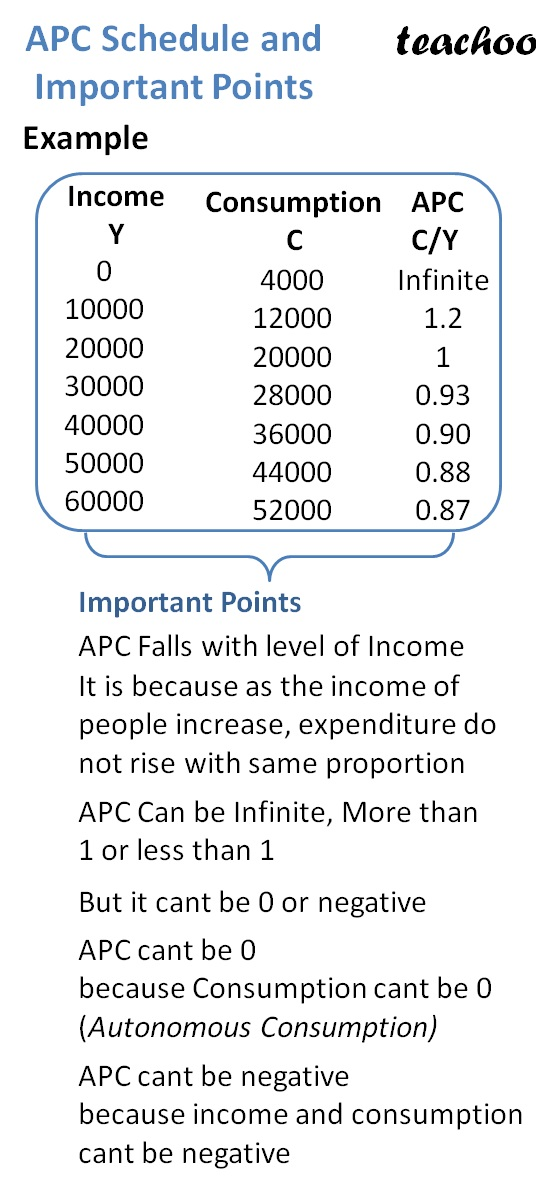APC Falls with level of Income

It is because as the income of people increase, expenditure do not rise with same proportion

When APC Is more than 1

It means Consumption Expenditure is more than Income

There are Dissavings in this case

When APC=1

It means Comsumption Expendiure = Income

It is Breakeven Point

When APC less than 1

It means Consumption Expenditure is less than Income

There is Savings in this case

APC Can never be 0

We know that APC = Consumption Exp/Income

APC Can be 0 only when Consumption expenditure is 0

Which is not possible because there is autonomous consumption expenditure

(There is minimum expenditure to be incurred even at 0 income)

Note

Lesser the APC, better it is for the economy

Developed Countries have lesser APC

Developing Countries have more APC

## How to Show APC on Graph

On X Axis, We represent Income

On Y Axis, We Represent Expenditure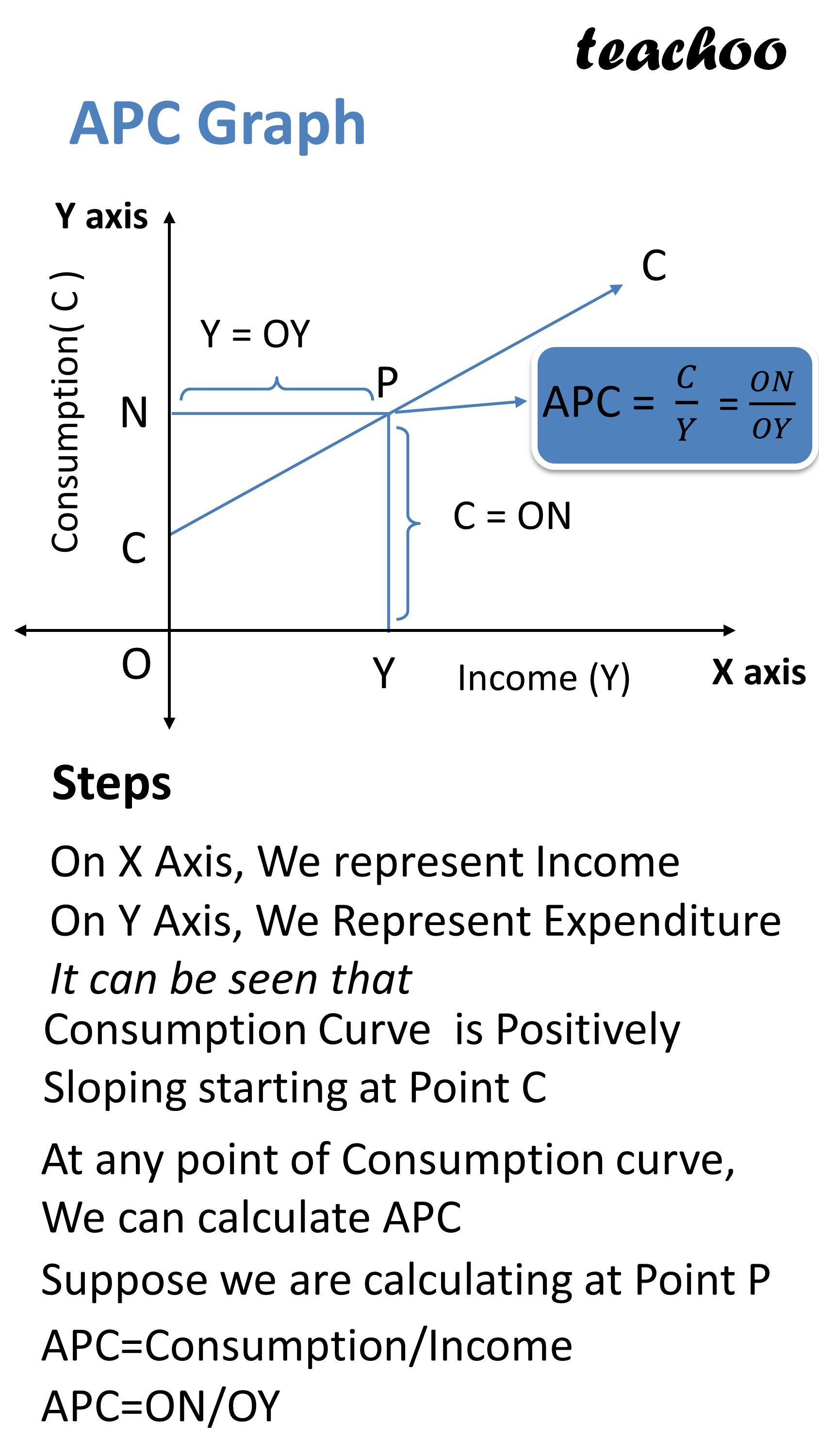It can be seen that

Consumption Curve is Positively Sloping starting at Point C

(It increases with increase in income)

At any point of Consumption curve, we can calculate APC

Suppose we are calculating at Point A

APC = Consumption/Income

APC = ON/OY

### NCERT Questions

No questions in this part

### Other Books

#### Question 1

What is APC?

State some important points about APC.

Learn in your speed, with individual attention - Teachoo Maths 1-on-1 Class

### Transcript

What is Average Propensity to Consume (APC) Suppose my Salary is Rs 50000 Out of this, I spend Rs 30000 Average Propensity to Consume =Consumption/Income =C/Y =30000/50000 =0.6 Income (Y) Consumption ( C ) What is APC? APC means Average Propensity to Consume It is Ratio of aggregate consumption expenditure to aggregate income APC=C/Y Does APC Always Remain Same? Income Y Consumption C APC C/Y 0 10000 20000 30000 40000 50000 60000 4000 12000 20000 28000 36000 44000 52000 Infinite 1.2 1 0.93 0.90 0.88 0.87 APC Falls with level of Income It is because as the income of people increase, expenditure do not rise with same proportion When is APC More than 1? Suppose my Salary is Rs 50000 But I spend Rs 60000 What is APC? Consumption ( C ) = Income (Y) = In this case, I am spending Rs 10000 from my pocket This is called Dissaving APC = Consumption/Income =60000/50000 =1.2 Income Y Consumption C APC C/Y APC is more than 1 when Consumption is more than income when Consumption is more than income When is APC =1? Suppose my Salary is Rs 50000 But I spend whole Rs 50000 What is APC? Consumption ( C ) = Income (Y) = In this case, I am not saving anything This is no Saving APC = Consumption/Income =50000/50000 =1 APC is equal to 1 when Consumption is equal to income There is no Savings in this case Can APC be 0? Example Suppose my Salary is Rs 50000 But I do not spend anything What is APC? Consumption ( C ) = Income (Y) = In this case, I am saving my full income Savings=Income=50000 APC = Consumption/Income =0/50000 =0 Income Y Consumption C APC C/Y APC is equal to 0 when there is 0 Consumption This is not possible due to autonomous consumption (There is some minimum consumption expenditure even at zero level of income) Can APC be Infinite? Example Suppose I am not working currently and my Salary is 0 I spend Rs 20000 on my household expenses What is APC? Consumption (C ) = Income (Y) = Even at 0 income, I have to incur some expenses This is called Autonomous Consumption Expenditure APC = Consumption/Income =20000/0 =Infinity APC is equal to infinity when there is 0 income This is possible due to autonomous consumption (There is some minimum consumption expenditure even at zero level of income) APC Schedule and Important Points Example Income Y Consumption C APC C/Y 0 10000 20000 30000 40000 50000 60000 4000 12000 20000 28000 36000 44000 52000 Infinite 1.2 1 0.93 0.90 0.88 0.87 Important Points APC Falls with level of Income It is because as the income of people increase, expenditure do not rise with same proportion APC Can be Infinite, More than 1 or less than 1 But it cant be 0 or negative APC cant be 0 because Consumption cant be 0 (Autonomous Consumption) APC cant be negative because income and consumption cant be negative APC Graph Steps On X Axis, We represent Income On Y Axis, We Represent Expenditure It can be seen that Consumption Curve is Positively Sloping starting at Point C At any point of Consumption curve, We can calculate APC Suppose we are calculating at Point P APC=Consumption/Income APC=ON/OY